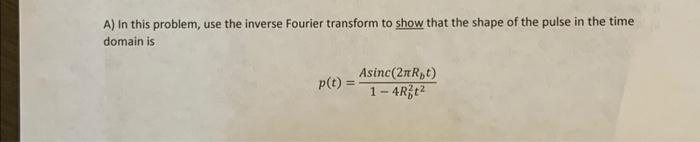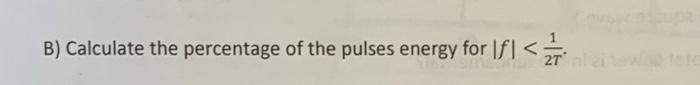# (Solved): just B please A) In this problem, use the inverse Fourier transform to show that the shape of the pu ...A) In this problem, use the inverse Fourier transform to show that the shape of the pulse in the time domain is $p(t)=\frac{A \operatorname{sinc}\left(2 \pi R_{b} t\right)}{1-4 R_{b}^{2} t^{2}}$ B) Calculate the percentage of the pulses energy for $$|f|<\frac{1}{2 T}$$.

We have an Answer from Expert